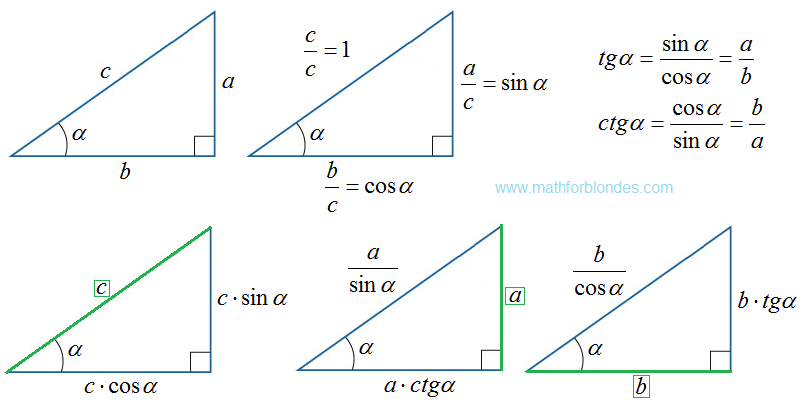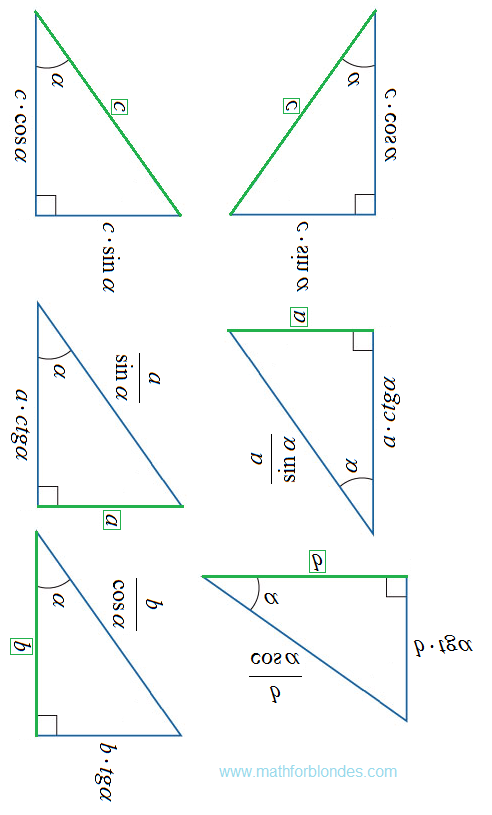## 1/31/2015

### Two angles of a triangle

Consider a very basic problem about two angles of a triangle are known. This problem sounds like this:

Two angles of a triangle are 53 degrees and 57 degrees. Find it the third corner of the triangle.

In any triangle all three angles. That is why the triangle is called. The value of the two angles of the three we know. Now I ask you a couple questions that will help solve this problem.

The first question. What is the sum of the angles of a triangle? This sacred knowledge of mathematics tease "A theorem on the sum of the angles of a triangle." As if they did not call it a law of nature, its essence does not change. Incidentally, the sum of the angles of a triangle belongs to the category of the mathematical knowledge that is easily stored for a long time, but that you never use not awake in their daily lives. Useless knowledge? No, but people use this knowledge is very limited range of professions, such as surveyors.Sum of the angles of a triangle

The second question. If you know that the sum of all the angles of a triangle is 180 degrees, with the arithmetic yourself cope? Here everything is simple. From the sum of the angles of a triangle 180 degrees subtract two prominent corner and get the value of the third angle of the triangle.

180 - 53 - 57 = 70 degrees

I do not want to show here ready-made solution, but ... First, the calculator have a lot of different buttons and accidentally be confused. In such cases, the scientists disappear satellites of Mars. So a complete solution for monitoring, can not hurt. Just check yourself.

Secondly, this is a very good opportunity to do what we do mathematics is strongly not recommended. We are taught to perform tasks with minimal downtime, and possibly without saving intermediate results. Actually, I did. On the one hand, it is correct. On the other hand, it does not give us the opportunity to understand, but what do we actually do?

Personally, I like to consider solving mathematical problems under the microscope in slow motion. Sometimes the impression is that we observe the focus by illusionist and all the secrets of the focus immediately crawl out. Let's look at the detailed solution of this problem on two well-known corners of the triangle and one unknown. Here's how it looks.Two angles of a triangle

And so. Someone measured the angles in a triangle is real. The measurements were performed only for the two corners. Man in high school and knows that the third angle can be simply calculated. This is the condition of the problem. Now, a detailed description of the meaning of the decision and the action carried out by us.

1. Write a law that establishes a relationship between the angles of a triangle, in algebraic form. I have already said that in mathematics it is called "A theorem on the sum of the angles of a triangle." The geometric shape of this law is shown in the first picture.

2. Transform the algebraic form of the law on the corners of a triangle to solve our specific problem.

3. Enter this formula in the data from the task ahead of us. Pass from the algebraic form to the physical.

4.Analiziruem physical model for solving the problem. Mathematical apparatus introduced the decimal system of numbers, other notations are absent. The physical device is represented by a measure of the degree angles, other angle units available. Only under these conditions we can perform addition and subtraction.

5. Go to the mathematical model of the physical problem and perform mathematical operations with numbers using a calculator, a sheet of paper or in your mind.

6. Get ready solution to the problem in physical form.

Here's a novel in verse about I turned to a very simple task. The accuracy of the description of this literary opus does not claim because the school did not teach me this, had to invent on the fly. All the described actions we perform automatically, without going into detailed explanations. I agree with mathematicians that stupid every solution of the problem in as much detail paint. But even more stupid stupid to perform the actions that you teach. In this case, the formation is converted into a conventional animal training.

## 1/20/2015

### Right triangle trigonometry

I will not tell you the rules and definitions of trigonometric functions on a right triangle. Mathematics is happy to do without me. I'll just show you a picture, which shows a right triangle trigonometry.Right triangle trigonometry

The top row shows who is who in the trigonometric zoo. Sine and cosine of the angle alpha - a relationship of legs to the hypotenuse. Tangent and cotangent - a relationship of legs. With hypotenuse usually no problem, and it is located opposite one of the right angle. But the legs as much as two and they are different. One is located in front of the angle alpha is called opposed (the picture side a). Another gently pressed against the corner called alpha and an adjacent (on the picture side b). Now, looking at the picture, you can easily formulate the definition of trigonometric functions on a right triangle.

The bottom row of images shows how to find the sides of a right triangle, if we know one side and the angle alpha. Known side is green. Using this side and trigonometric functions, you can easily find the other two sides of a right triangle.

Spin this picture as you like, turn face down and look up to the light - trigonometric dependence in a right triangle from it are not changed.Spin this picture

This picture you can come in handy in the future, the study of physics, theoretical mechanics, in engineering calculations. By the time you've forgotten how to define and use trigonometric functions in a right triangle.

And the saddest thing in the end. If you teach mathematics to use mathematics, these pictures you drew to themselves for a few minutes, without any textbooks. After all this is done simply elementary.

## 1/17/2015

### Hilbert's sixth problem

Here I have in the comments appeared one modest request:

Help solve the problem associated with the extend of Kronecker–Weber theorem on abelian extensions of the rational numbers to any base number field.

Such a number of clever words in one sentence, I have not met. I immediately suspected that not all of the school textbook is taken, and from some other place. Type in the search phrase Wikipedia "Kronecker–Weber theorem" and look at the result. That's right, I slipped twelfth problem of Hilbert, has not been solved so far. Suddenly her uncle decides - all would be funny!

You should not expect. For me, the phrase "abelian extensions", "the rational numbers to any base number field" is no more than a collection of letters. In higher mathematics, I did not go through dog training and have no idea what to do, when he heard these phrases. Moreover, I do not even have a clue what the root of the unit n-th degree is different from the unit itself. In mathematics, the same thing can have different names, the same name can mean a variety of things. Hence, problems arise.

More than a hundred years ago David Hilbert formulated twenty-three problems of mathematics. Some problems have been resolved, some partially solved two problems remain unsolved to this day. There are also a number of problems that are simply hushed for clarity: "too vague" or "requires clarification of the phrase". Among these "jammed" caught my attention problems Hilbert's sixth problem, which is: Mathematical treatment of the axioms of physics. Mathematics jumped from the solution of this problem with the phrase "too vague". This is where I disagree with them.

Formulation of Hilbert very clearly, that's just to express nothing - not taken root in physics ideas about mathematics axioms, as his time in the chemistry did not take the idea of negative numbers. This mathematics all their theories verify with the axioms of physics as his theories verify with the experimental results. There physics postulates, but it's just a temporary patch on the white spots of our knowledge. Sooner or later, the postulates are replaced by physical laws. Temporary postulates physicists do not go to any comparison with the monumental firmness axioms of mathematics.

Here and there is a very sudden decision Hilbert's sixth problem - the language of mathematics is much easier to state "axioms" of religion than the axioms of physics. Looks mathematical presentation of the fundamentals of religion something like this:

- Sacred texts in religion - axioms and definitions in mathematics;

- All that is in this world created by God - in mathematics, the phrase "Let us given ..." by default assumes that everything God gives us;

- The story of Noah's ark and the "pair of every creature" - set theory;

- Man is composed of body and soul - complex numbers consist of real and imaginary parts;

- Kingdom of God - a complex space;

- God and the devil, good and evil - the positive and negative numbers;

- Holy Cross - Cartesian coordinate system.

If desired, you can thoroughly examine the sacred texts of religion and the sacred texts of Mathematicians (axioms and definitions) in search of other matches. In the language of religion, math is pretty good presents.

In my opinion, the problems in modern mathematics and believers alike - autism. They live in an imaginary world and not pay attention to their surroundings. Teaching of mathematics is very similar to the missionary preaching - we need to learn and do what they say preachers. All attempts to draw attention to the preachers of the surrounding reality ends are being sent to the sacred texts: "Read the Bible", "Read the definition."

## 1/07/2015

### Happy New Year!Happy New Year!

Happy New Year to you all! Do not look at the numbers in 2015, they may look much prettier. It all depends on what we want to see them.2015 year

The number 2015 in the binary system looks much nicer and has an almost perfect symmetry. But it will look like a calendar for 2015 in the binary system.Calendar for 2015

And New Year's letter to Santa Claus. To the Russian Santa Claus (Ded Moroz) from Ukrainian girl whose father was killed in the Russian-Ukrainian war. No comment.New Year's letter to Santa Claus from Ukrainian girl

"Good afternoon, Santa Claus!
I have two big dreams.
My first dream is to end the war and did not die, our Pope!
Second, I want the laptop to communicate with children who also lost their dads, and who also want peace.
Thank you for understanding.Happy New Year from PutinHumanitarian assistance from Putin in Ukraine